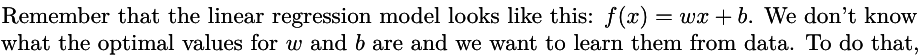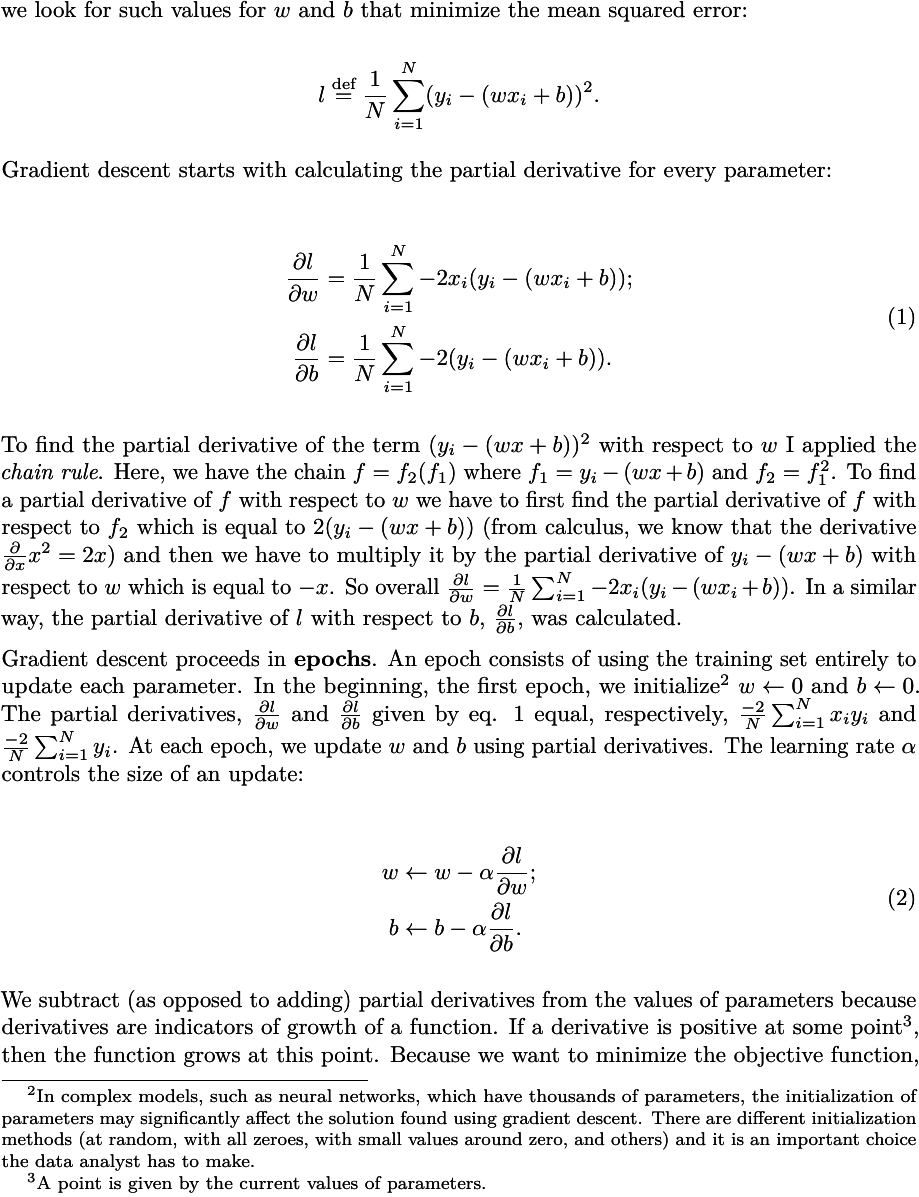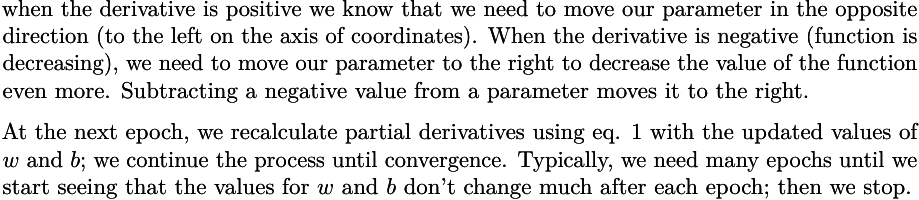# on 21-Oct-2020 (Wed)

#### Annotation 4763933609228

 #MLBook #expectation #expected-value #machine-learning #statistics Let a discrete random variable $$X$$ have $$k$$ possible values $$\{ x_i \}_{i=1}^k$$. The expectation of $$X$$ denoted as $$\mathbb E[X]$$ is given by, \begin{align} \mathbb E[X] & \stackrel{\textrm{def}}{=} \sum_{i=1}^k \left[ x_i \cdot \textrm{Pr} \left( X = x_i \right) \right] \\ & = x_1 \cdot \textrm{Pr} \left( X = x_1 \right) + x_2 \cdot \textrm{Pr} \left( X = x_2 \right) + \cdots + x_k \cdot \textrm{Pr} \left( X = x_k \right) \end{align} where $$\textrm{Pr} \left( X = x_i \right)$$ is the probability that $$X$$ has the value $$x_i$$ according to the pmf. The expectation of a random variable is also called the mean, average or expected value and is frequently denoted with the letter $$\mu$$ . The expectation is one of the most important statistics of a random variable.

#### pdf

cannot see any pdfs

#### Annotation 5969688792332

 [unknown IMAGE 5969687481612] #dynamics #engineering-problems #has-images #numerical-accuracy #statics #vector-mechanics

#### pdf

cannot see any pdfs

#### Annotation 5969692986636

 [unknown IMAGE 5969691675916] #calculations #dynamics #engineering-mechanics #has-images #statics

#### pdf

cannot see any pdfs

#### Annotation 5998197214476

 [unknown IMAGE 5998195117324] #MLBook #has-images #logistic-regression #machine-learning #neural-network #unit

#### pdf

cannot see any pdfs

#### Annotation 5998204030220

 #MLBook #epocs #gradient-descent #has-images #machine-learning